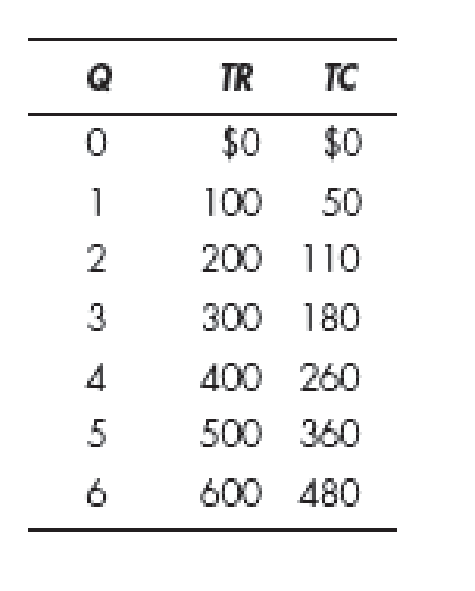Chapter 22, Problem 3WNG

Chapter
Section
Textbook Problem

According to the accompanying table, what quantity of output should the firm produce? Explain your answer.To determine

Quantity of output produced by the firm.

Explanation

Table 1 shows the different quantities of output produced at different total revenues and total costs.

Table 1

 Quantity Total revenue Total cost 0 $0$0 1 100 50 2 200 110 3 300 180 4 400 260 5 500 360 6 600 480

From the different quantitates shown in Table 1, the firm should produce 5 units of output because the total revenue is greater than the total cost, and also at quantity 5, the marginal revenue is equal to the marginal cost. The calculation of marginal revenue and marginal cost is shown below:

Marginal revenue can be calculated as follows:

Marginal revenue=Total revenuePresentTotal revenuePreviousQuantityPresentQuantityPrevious        (1)

Substitute the respective values in Equation (1) to calculate the marginal revenue at quantity 5 units

Still sussing out bartleby?

Check out a sample textbook solution.

See a sample solution

The Solution to Your Study Problems

Bartleby provides explanations to thousands of textbook problems written by our experts, many with advanced degrees!

Get Started

List and describe six costs of inflation.

Principles of Economics (MindTap Course List)

Why is productivity important?

Principles of Microeconomics (MindTap Course List)

SPOT AND FORWARD RATES Anderson Australian Imports has agreed to purchase 15,000 cases of Australian wine for 4...

Fundamentals of Financial Management, Concise Edition (with Thomson ONE - Business School Edition, 1 term (6 months) Printed Access Card) (MindTap Course List)